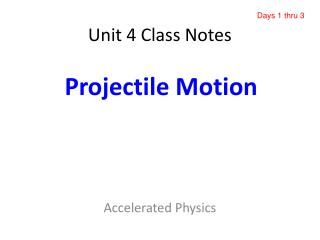# Unit 4 Class Notes - PowerPoint PPT PresentationDownload PresentationUnit 4 Class Notes

Unit 4 Class NotesDownload Presentation## Unit 4 Class Notes

- - - - - - - - - - - - - - - - - - - - - - - - - - - E N D - - - - - - - - - - - - - - - - - - - - - - - - - - -
##### Presentation Transcript

1. Days 1 thru 3 Projectile Motion Unit 4 Class Notes Accelerated Physics

2. Day #1 Free-Falling Object Review

3. In-Class Practice

4. In-Class Practice

5. In-Class Practice

6. In-Class Practice

7. In-Class Practice

8. In-Class Practice

9. In-Class Practice

10. Day #2 Horizontal Projectiles

11. From Reading Assignment handout

12. Horizontal Projectiles • The first example on the previous slide is often called a horizontal projectile. This is the first example we will look at. • The object is given an initial horizontal velocity. Thus, velocity is purely horizontal (no y component) • Our initial velocity in the y direction viy=0. • Next, analyze the motion in the horizontal and vertical direction separately.

13. Figure #3 Figure #2 Figure #1 From Reading Assignment handout

14. Using figures from previous slide • Figure 1 – Free fall object. The object’s position after every second of falling is shown. The object is accelerating because it travels a longer distance in each successive time interval. • Figure 2 – Horizontally traveling object at a constant velocity. Acceleration equals zero so each time interval shows the same distance traveled. • Figure 3 – A combination of both these motions. When a projectile falls, it accelerates downward so its y direction matches figure 1. However, since nothing (including air) slows the object down as it moves horizontally, its x direction matches figure 2.

15. Since projectile motion is a combination… • We will analyze the motion separately. The only shared variable between the two motions is TIME (since they both occur in the same amount of time) • Solve for time in vertical (y) side, then plug in to horizontal side.

16. There are so few equations in the x direction because a=0 so velocity is constant. • In the y direction we have the 4 familiar acceleration formulas. • EXAMPLE 1: A ball is rolled off a flat roof hat is 30 m above the ground. If the ball’s initial speed is 15 m/s, find the time needed to strike the ground as well as the distance that it lands away from the building. Horizontal (x) Vertical (y) From Reading Assignment handout

17. Horizontal (x) Vertical (y) From Reading Assignment handout

18. Horizontal (x) Vertical (y) From Reading Assignment handout

19. In-Class Practice

20. In-Class Practice

21. In-Class Practice

22. Day #3 COMPETITION LAB!!!! (Ball Rolling Off Table)

23. Day #4 Tracking and Impact Velocity

24. Side-ways Toss (Gravity Turned Off) Each position corresponds to 1 sec later than the previous position (starting with the red dot)

25. Side-ways Toss (Gravity Turned Off) Horizontal Projectiles Free-Fall

26. Impact Velocity Side-ways Toss (Gravity Turned Off) Horizontal Projectile Free-Fall Velocities

27. Impact Velocity Vfx q Vfy Vimpact

28. From Reading Assignment handout

29. Horizontal (x) Vertical (y) 4 m/s  19.6 m/s V2 V2 = 20 m/s [78.46o BH] From Reading Assignment handout

30. From Reading Assignment handout

31. In-Class Practice

32. In-Class Practice

33. In-Class Practice

34. In-Class Practice

35. In-Class Practice

36. In-Class Practice

37. Day #5 Angled Projectiles on Flat Ground

38. What we’ve done so far What we still need to do

39. Side-ways Toss (Gravity Turned Off) Throw-up, then Free-Fall Projectiles at Angles

40. So, what are the Equations we use for this “NEW” type of projectile???? Horizontal Vertical There are NO NEW EQUATIONS 

41. vi viy q vix vi So…..How are “angled” projectiles different than “horizontal” projectiles? q Viy = 0 (for horizontal projectiles) = visinq Viy = something, + or - (for horizontal projectiles) = vicosq

42. q Vix = Vfx = Vx = 0 (since ax = 0) Vy, top = 0 When rising, Vy is positive (when falling = Vy is negative) The velocity at every moment in time is the resultant of the two velocity components If launching and landing occurs at the same height (on level ground) then the launching/landing speeds & angles are the same What are some important things to remember? vi q

43. In-Class Practice

44. In-Class Practice

45. In-Class Practice

46. In-Class Practice

47. In-Class Practice

48. Day #6 MORE Angled Projectiles … Sometimes NOT on Flat Ground

49. In-Class Practice

50. In-Class Practice